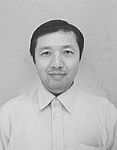GIGA Yoshikazu

GIGA YoshikazuTitle Professor Department of Mathematics (UG), Graduate School of Mathematical Sciences309, Graduate School of Mathematical Sciences Bldg. 3F, Komaba Campus +81-3-5465-7023　|　47023（ext.）

## Research Field

Nonlinear Analysis

## Research Subject

Mathematical Analysis on Differential Equations descrbing Nonlinear Diffusive Phenomena

## Current Research

Various differential equations have been proposed with the purpose to model phenomena in nature such as motion of fluid, crystal growth and to handle problems in technology for example in image processing. My major research topic is nonlinear diffusion equations which form an important class of partial differential equations. ( A partial differential equation is a differential equation having several independent variables.) My particular interest lies in solvability of partial differential equations under given conditions and in tracking behavior of solutions in a rigorous mathematical framework even if solutions develop singularities.

## Keywords

Navier-Stokes equations, surface evolution equations, blow-up phenomena of solutions, viscosity solutions, variational analysis,nonlinear diffusion equations